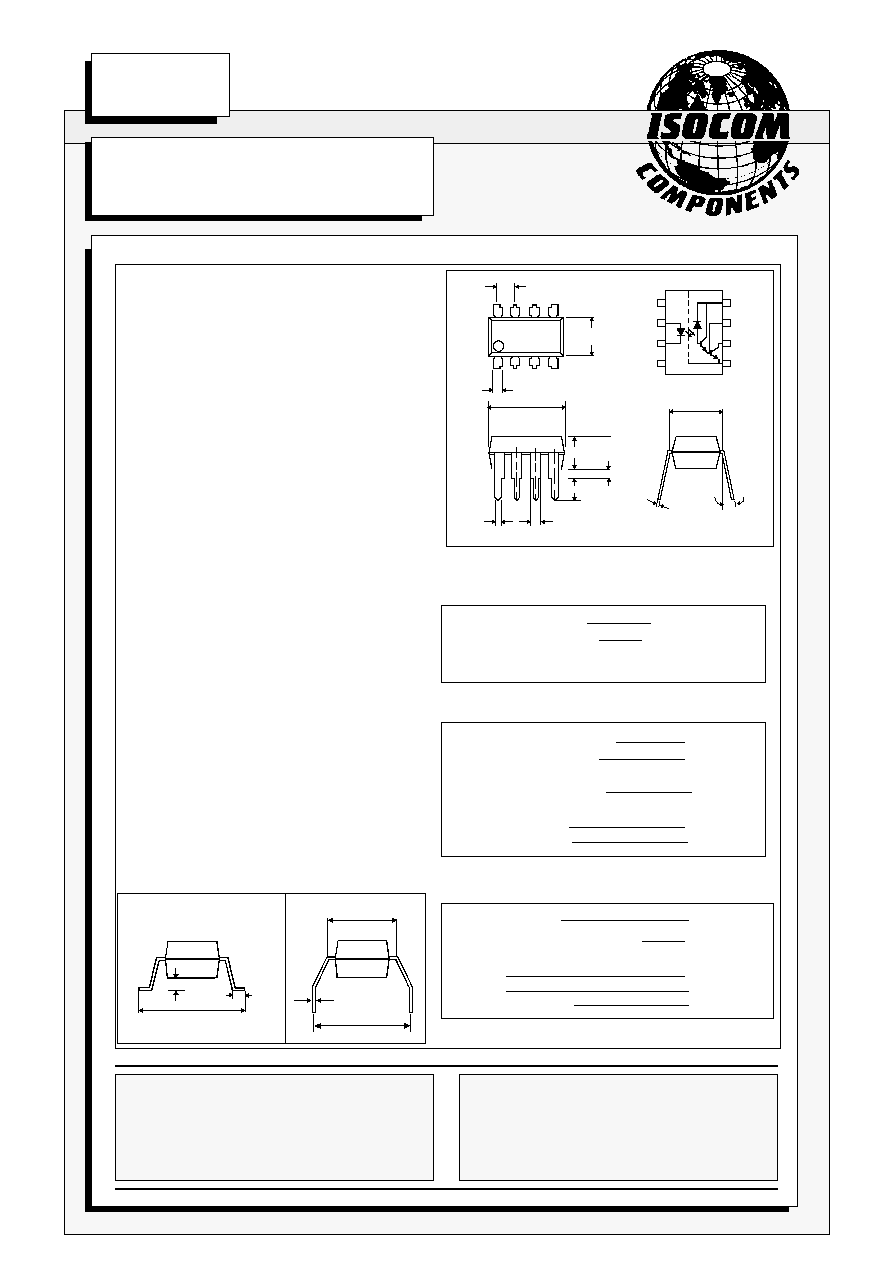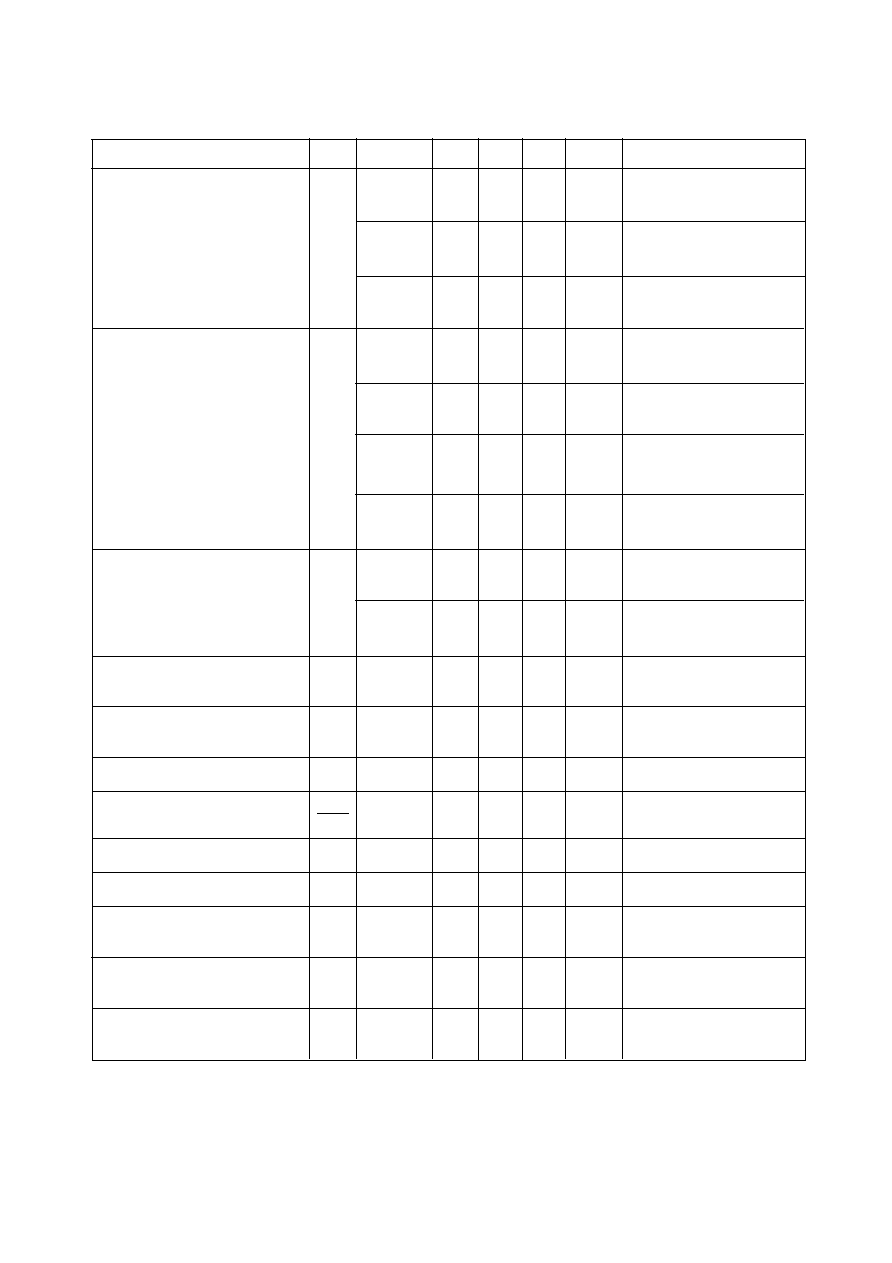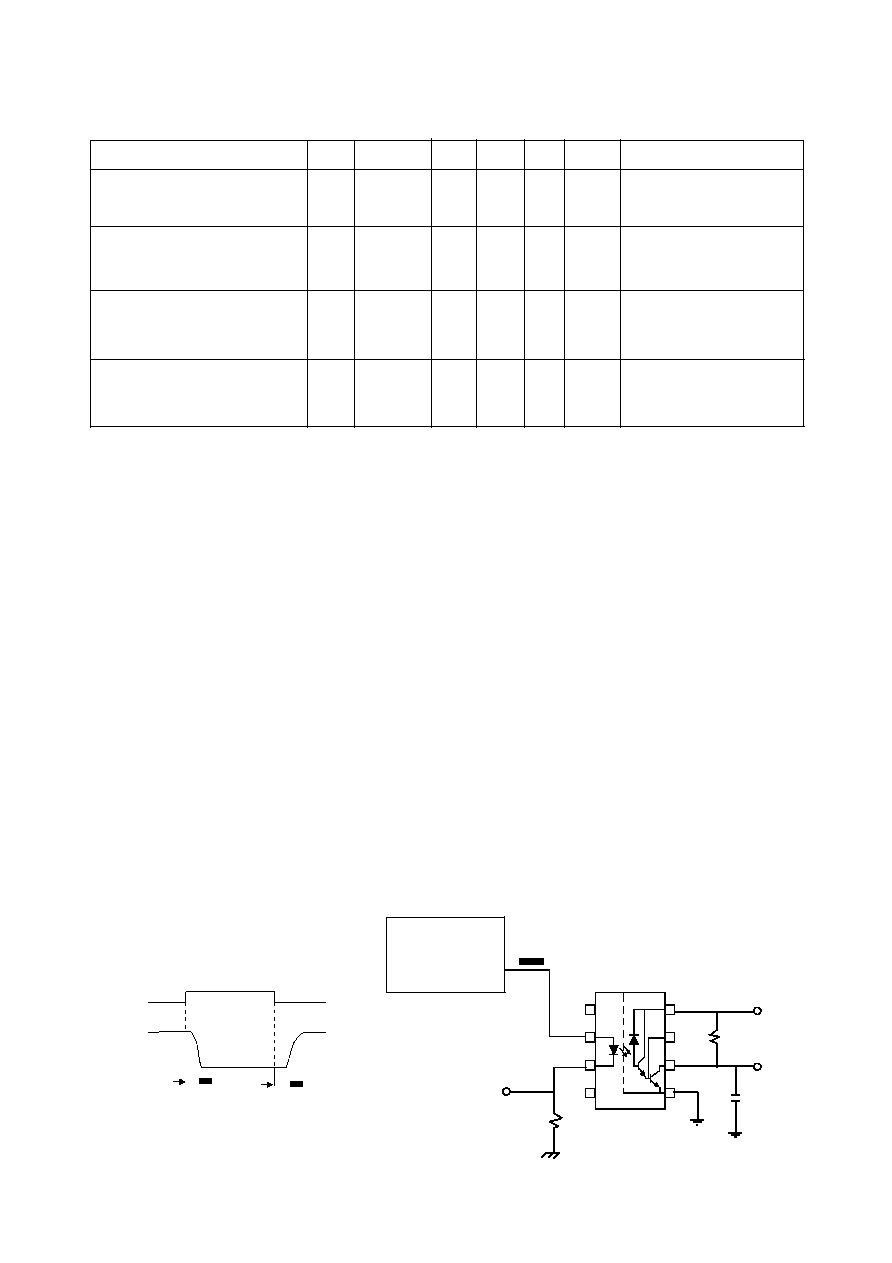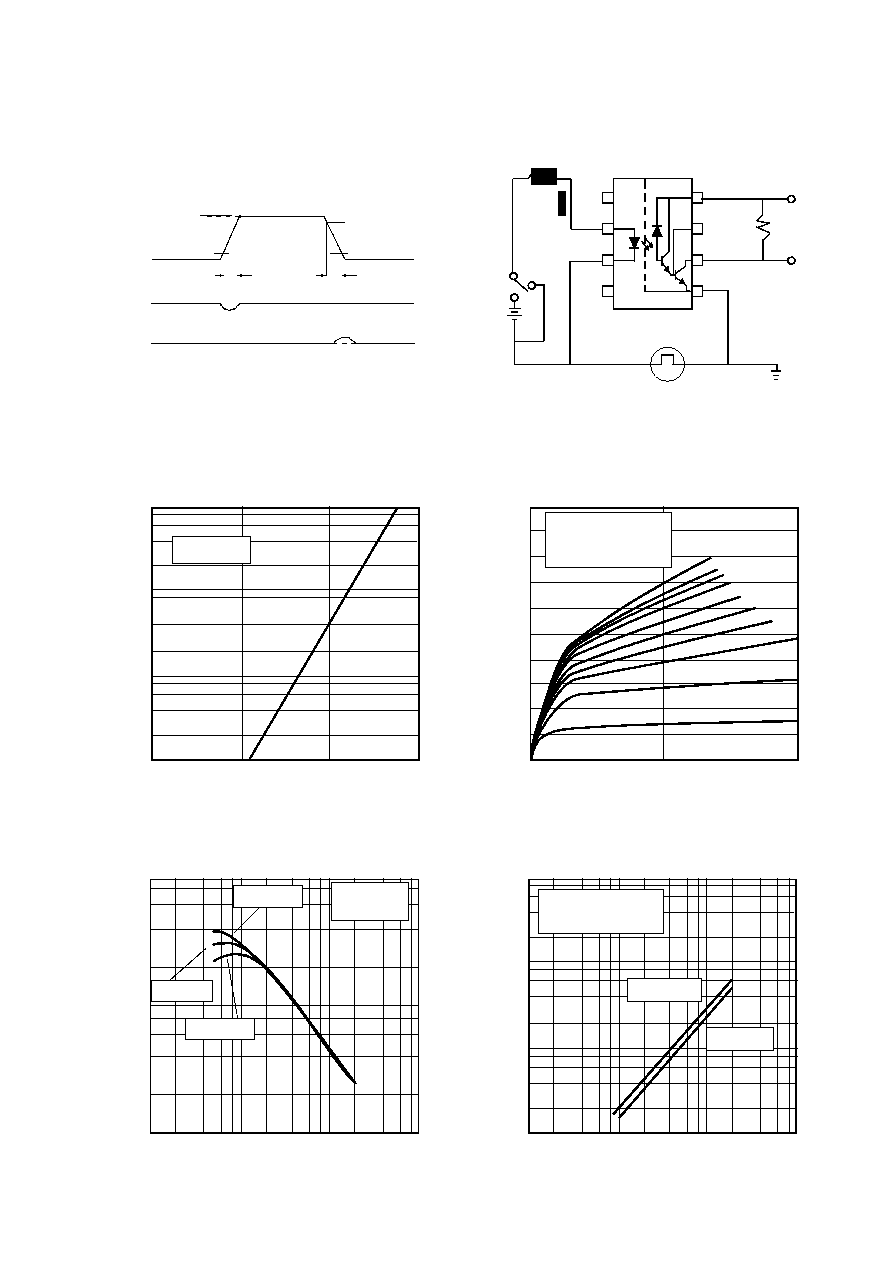Search datasheet (1.687.043 components) Search fieldPart namePart descriptionAbout site Manufacturers list ChipFindIC search engine AllXrefCross-reference database### Datasheet: 6N138 (Isocom Incorporated)

Single Channel, High Gain
Download:PDFZIPISOCOM COMPONENTS LTD
Unit 25B, Park View Road West,
Park View Industrial Estate, Brenda Road
Hartlepool, Cleveland, TS25 1YD
Tel: (01429) 863609 Fax :(01429) 863581
22/5/03
DB91037-AAS/A3
6N138, 6N139
HIGH SPEED OPTICALLY
COUPLED ISOLATOR
PHOTODARLINGTON OUTPUT
ABSOLUTE MAXIMUM RATINGS
(25°C unless otherwise specified)
Storage Temperature
-55°C to + 125°C
Operating Temperature
-40°C to + 85°C
(1/16 inch (1.6mm) from case for 10 secs) 260°C
INPUT DIODE
Average Forward Current
20mA ( 1 )
Peak Forward Current
40mA
( 50% duty cycle, 1ms pulse width )
Peak Transient Current
1.0A
(
1
µ
s P.W., 300 pps)
Reverse Voltage
5V
Power Dissipation
35mW( 2 )
DETECTOR
Output Current
60mA( 3 )
Emitter-base Reverse Voltage
0.5V
Supply and Output Voltage
6N138
-0.5 to +7V
6N139
-0.5 to +18V
Power Dissipation
100mW (4 )
APPROVALS
l
UL recognised, File No. E91231
DESCRIPTION
These diode-darlington optocouplers use a light
emitting diode and an integrated high gain photon
detector to provide 2500Volts
RMS
electrical isolation
between input and output. Seperate connection for
the photodiode bias and output darlington collector
improve the speed up to a hundred times that of a
conventional photo-darlington coupler by reducing
the base-collector capacitance.
FEATURES
l
High speed - DC to 300kBits/s operation
l
High Common Mode Transient
Immunity 10kV/
µ
s typical
l
TTL Compatible - 0.1V V
OL
typical
l
Base access allows Gain Bandwidth
l
Low Input Current Requirement - 0.5mA
l
High Current Transfer Ratio - 1100% typ.
l
Open Collector Output
l
2500V
RMS
Withstand Test Voltage, 1 min
l
6N139 has improved noise shield which
gives superior common mode rejection
l
Options :-
Surface mount - add SM after part no.
Tape&reel - add SMT&R after part no.
l
All electrical parameters 100% tested
l
Custom electrical selections available
APPLICATIONS
l
l
Digital logic ground isolation
l
Telephone ring detector
l
0.3
0.5
Dimensions in mm
6.9
6.3
1.3
15°
Max
3.3
4.0
3.6
2.54
9.7
9.1
0.5
1.3
* 6N139 NOISE SHIELD
7.62
4
3
2
1
8
7
6
5
*
OPTION G
OPTION SM
10.16
10.2
9.5
0.3
1.2
0.6
1.4
0.9
7.62
SURFACE MOUNT
ISOCOM INC
1024 S. Greenville Ave, Suite 240,
Allen, TX 75002 USA
Tel: (214) 495-0755 Fax: (214) 495-0901
e-mail info@isocom.com
http://www.isocom.comDB91037-AAS/A3
22/5/03
ELECTRICAL CHARACTERISTICS ( T
A
= 0°C to 70°C Unless otherwise noted )
PARAMETER
SYM DEVICE
MIN TYP* MAX UNITS TEST CONDITION
6N139
400
1100
%
I
F
= 0.5mA,V
O
= 0.4V
V
CC
= 4.5V
6N139
500
1300
%
I
F
= 1.6mA, V
O
= 0.4V
Current Transfer Ratio
CTR
V
CC
= 4.5V
(note 5, 6)
6N138
300
1300
%
I
F
= 1.6mA, V
O
= 0.4V
V
CC
= 4.5V
6N139
0.1
0.4
V
I
F
= 1.6mA, I
O
= 8mA
V
CC
= 4.5V
6N139
0.1
0.4
V
I
F
= 5mA, I
O
= 15mA
Logic Low Output Voltage
V
OL
V
CC
= 4.5V
(note 6)
6N139
0.1
0.4
V
I
F
= 12mA, I
O
= 24mA
V
CC
= 4.5V
6N138
0.1
0.4
V
I
F
= 1.6mA, I
O
= 4.8mA
V
CC
= 4.5V
6N139
0.1
100
µ
A
I
F
= 0mA
Logic High Output Current
I
OH
V
O
= V
CC
= 18V
(note 6)
6N138
0.01
250
µ
A
I
F
= 0mA
V
O
= V
CC
= 7V
Logic Low Supply Current
I
CCL
0.4
1.5
mA
I
F
= 1.6mA,V
O
= open
(note 6)
V
CC
= 18V
Logic High Supply Current
I
CCH
0.05
10
µ
A
I
F
= 0mA,V
O
= open
(note 6)
V
CC
= 18V
Input Forward Voltage
V
F
1.45
1.7
V
I
F
= 1.6mA, T
A
= 25°C
Temperature Coefficient
V
F
-1.8
mV/°C
I
F
= 1.6mA
of Forward Voltage
T
A
Input Reverse Voltage
V
R
5
V
I
R
= 10
µ
A,T
A
= 25°C
Input Capacitance
C
IN
60
pF
f
= 1MHz, V
F
= 0
Input-output Isolation Voltage
V
ISO
2500
5000
V
RMS
R.H.equal to or less than
50%, t = 1min. T
A
= 25°C
Resistance (Input to Output)
R
I-O
10
12
V
I-O
= 500V dc
(note 7)
Capacitance (Input to Output)
C
I-O
0.6
pF
f = 1MHz
(note 7)
* All typicals at T
A
= 25°CSWITCHING SPECIFICATIONS AT T
A
= 25°C ( V
CC
= 5V Unless otherwise noted )
PARAMETER
SYM DEVICE
MIN TYP MAX UNITS TESTCONDITION
Propagation Delay Time
6N139
5.0
25
µ
s
I
F
= 0.5mA,R
L
= 4.7k
to
Logic Low at Output
t
PHL
6N139
0.2
1
µ
s
I
F
= 12mA,R
L
= 270
( fig 1 )( note6,8 )
6N138
1.0
10
µ
s
I
F
= 1.6mA,R
L
= 2.2k
Propagation Delay Time
6N139
1.0
60
µ
s
I
F
= 0.5mA,R
L
= 4.7k
to
Logic High at Output
t
PLH
6N139
1.0
7
µ
s
I
F
= 12mA,R
L
= 270
( fig 1 )( note6,8 )
6N138
4.0
35
µ
s
I
F
= 1.6mA,R
L
= 2.2k
Common Mode Transient
Immunity at Logic High
CM
H
1000 10000
V/
µ
s
I
F
= 0mA, V
CM
= 10V
PP
Level Output ( fig 2 )( note9 )
R
L
= 2.2k
Common Mode Transient
Immunity at Logic Low
CM
L
1000 10000
V/
µ
s
I
F
= 1.6mA,V
CM
= 10V
PP
Level Output ( fig 2 )( note9 )
R
L
= 2.2k
NOTES:-
1.
Derate linearly above 50
o
C free air temperature at a rate of 0.4 mA/°C.
2.
Derate linearly above 50
o
C free air temperature at a rate of 0.7 mW/°C.
3.
Derate linearly above 25
o
C free air temperature at a rate of 0.7 mA/°C.
4.
Derate linearly above 25
o
C free air temperature at a rate of 2.0 mW/°C.
5.
CURRENT TRANSFER RATIO is defined as the ratio of output collector current,I
O
, to the forward LED
input current, I
F
multiplied by 100%.
6.
Pin 7 open.
7.
Device considered a two-terminal device: pins 1,2,3, and 4 shorted together and pins 5,6,7 and 8 shorted
together.
8.
Use of a resistor between pin 5 and 7 will decrease gain and delay time.
9.
Common mode transient immunity in Logic High level is the maximum tolerable (positive) dVcm/dt on
the leading edge of the common mode pulse V
CM
to assure that the output will remain in a Logic High
state (i.e. V
O
> 2.0V). Common mode transient immunity in Logic Low level is the maximum tolerable
(negative) dVcm/dt on the trailing edge of the common mode pulse signal, V
CM
to assure that the output
will remain in Logic Low state (i.e. V
O
< 0.8V).
FIG.1 SWITCHING TEST CIRCUIT
2
I
F
Monitor
0
I
F
V
O
1.5V
100
1.5V
5V
t
PHL
t
PLH
V
OL
R
L
V
O
C
L
= 15pF
8
7
6
5
PULSE
GENERATOR
Z
O
= 50
t
r
= 5ns
I
F
1
4
3
10% Duty Cycle
1/f < 100
µ
s
5V
DB91037-AAS/A3
22/5/03DB91037-AAS/A3
22/5/03
FIG. 2 TEST CIRCUIT FOR TRANSIENT IMMUNITY AND TYPICAL WAVEFORMS
1
3
4
+
-
A
PULSE GEN.
V
CM
0V
V
CM
I
F
V
FF
5V
5V
V
OL
V
O
V
O
SWITCH AT A: I
F
= 0mA
SWITCH AT B: I
F
= 1.6mA
10V
10%
90%
10%
90%
t
r
t
f
B
8
7
6
5
2
R
L
V
O
Output current
I
O
(mA)
Output Current vs.
Output Voltage
1.0 1.2 1.4 1.6
Forward current
I
F
(mA)
Forward Current vs. Forward
Voltage
Forward voltage V
F
(V)
Current Transfer Ratio vs.
Forward Current
Current transfer ratio CTR (%)
Forward current I
F
(mA)
0.1 1.0 10 100
Supply current
I
CCL
(mA)
V
O
= 0.4V
V
CC
= 5V
0 1.0 2.0
Output voltage V
O
(V)
Supply Current vs.
Forward Current
0.01
0.1
0.02
0.04
0.2
0.4
1
2
4
10
T
A
= 25°C
T
A
= 0°C
T
A
= -40°C
T
A
= 85°C
400
200
100
1000
2000
4000
10000
6N139 V
CC
= 18V
6N138 V
CC
= 7V
V
CC
= 18V
V
CC
= 7V
Forward current I
F
(mA)
0.1 1.0 10 100
0.1
0.2
0.4
1
2
4
10
20
40
100
V
CC
= 5V
T
A
= 25°C
I
F
= 0.5mA / step
0
10
20
30
40
50
60
70
80
90
100© 2020 • ICSheet• Contact form• Main page